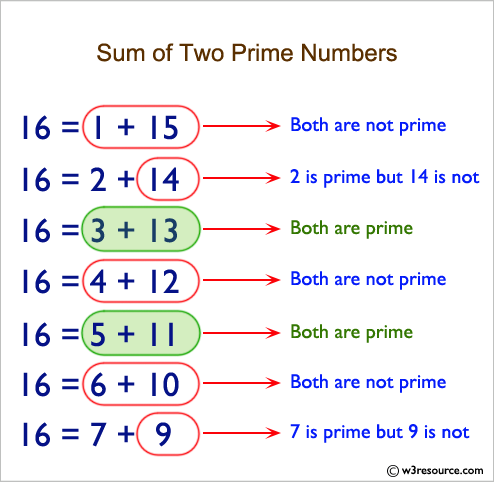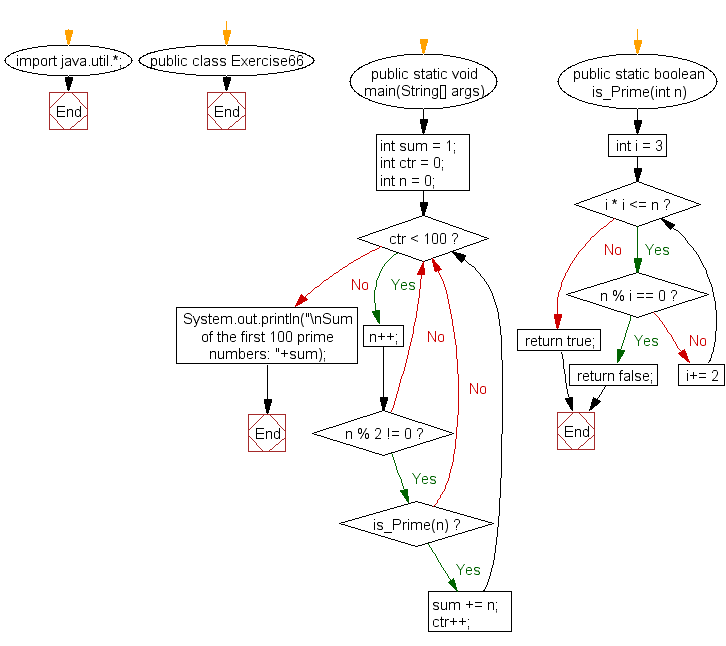﻿ Java exercises: Compute the sum of the prime numbers till 100 - w3resource# Java Exercises: Compute the sum of the prime numbers till 100

## Java Basic: Exercise-66 with Solution

Write a Java program to Compute the sum of the prime numbers till 100.

Pictorial Presentation:Sample Solution:

Java Code:

``````import java.util.*;
public class Exercise66 {
public static void main(String[] args)
{
int sum = 1;
int ctr = 0;
int n = 0;

while (ctr < 100) {
n++;
if (n % 2 != 0) {
// check if the number is even
if (is_Prime(n)) {
sum += n;
}
}
ctr++;
}
System.out.println("\nSum of the prime numbers till 100: "+sum);
}

public static boolean is_Prime(int n) {
for (int i = 3; i * i <= n; i+= 2) {
if (n % i == 0) {
return false;
}
}
return true;
}
}
```
```

Sample Output:

```Sum of the prime numbers till 100: 1060
```

Flowchart:Java Code Editor:

What is the difficulty level of this exercise?

Test your Programming skills with w3resource's quiz.

﻿

## Java: Tips of the Day

countOccurrences

Counts the occurrences of a value in an array.

Use Arrays.stream().filter().count() to count total number of values that equals the specified value.

```public static long countOccurrences(int[] numbers, int value) {
return Arrays.stream(numbers)
.filter(number -> number == value)
.count();
}
```

Ref: https://bit.ly/3kCAgLb